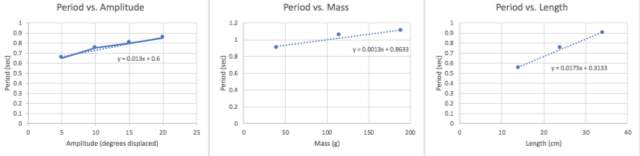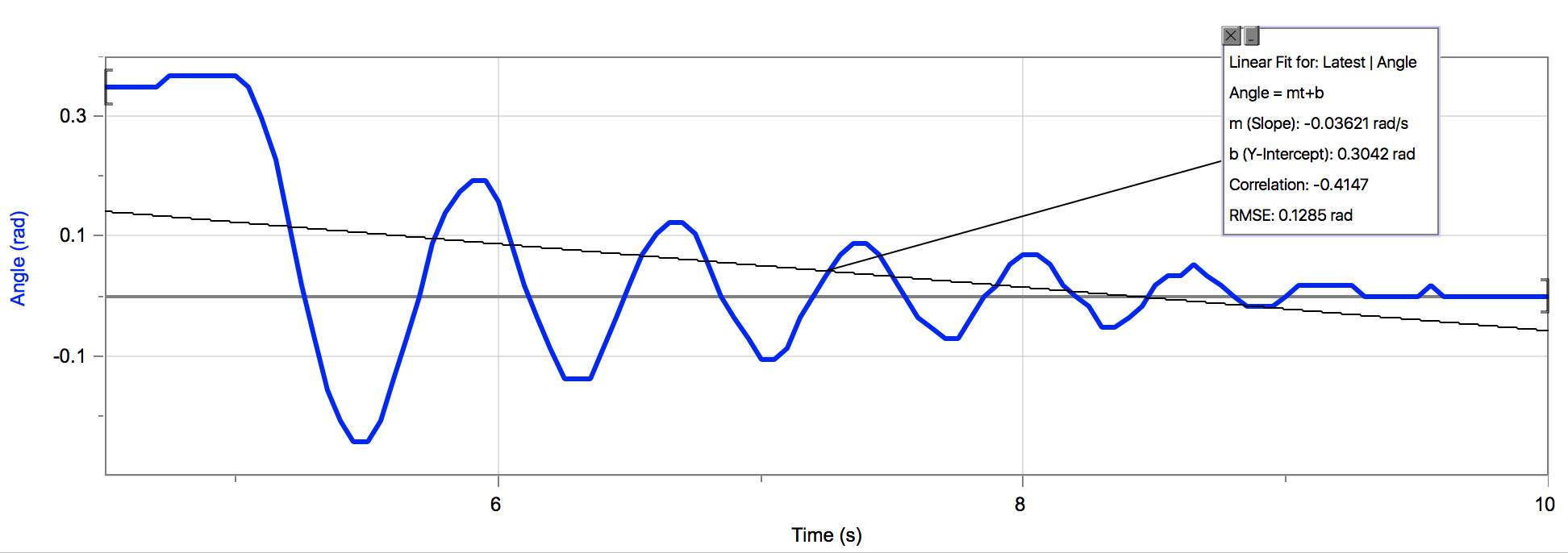Disclaimer: This is an example of a student written essay.

Any scientific information contained within this essay should not be treated as fact, this content is to be used for educational purposes only and may contain factual inaccuracies or be out of date.

# Simple Harmonic Motion of a Pendulum

 ✅ Paper Type: Free Essay ✅ Subject: Physics ✅ Wordcount: 2263 words ✅ Published: 11th May 2021

Introduction

This lab aims to explore the concept of periodic motion, which is a type of motion that repeats itself in a regular way. The time it takes for an object to complete one full motion, known as a cycle, is called the period (T). This lab discusses two examples of periodic motion, an oscillating pendulum and an oscillating mass on the end of a spring. When an object is stationary and oscillating it represents motion that is periodic in time, where its position (y) is a function of time (t), otherwise known as y(t). However, when an object is moving and oscillating, the position of the object (y) depends on the horizontal position (x), producing the function y(x,t). This is the behavior shown by a travelling wave, however, it was not discussed in this lab.

In experiment 1, simple harmonic motion is measured in a physical pendulum. Experiment 2 measures simple harmonic motion using a spring. The equation for a pendulum that relates the variables involved is:

where frequency f the inverse of period T, $f=\frac{1}{T}$. Therefore: where I = (1/3)mr², so . In a simple pendulum, moment of inertia is I=mr², so . When incorporating angle displaced, the equation is .

Experiment 1: the Pendulum

In this experiment, displacement of  a mass hanging from a swinging pendulum was measured via a motion detector under varying conditions. In the first portion, amplitude was manipulated. In the second, the mass of the hanging weight was manipulated. In the third, the length of the pendulum is manipulated (center of mass to the axis of rotation).

Data Analysis:

 Trial # Amplitude (degrees) Period (s) Expected period based on equation % difference 1 5 0.65 0.34 62.62 2 10 0.75 0.49 41.93 3 15 0.80 0.60 28.57 4 20 0.85 0.68 22.22

Table 1. Period vs. Amplitude with mass = 40g, length = 34cm

 Trial # Mass (g) Period (s) Expected period (s) based on equation$T=2\pi$ % difference 1 40 0.9 1.17 26.09 2 115 1.05 1.17 10.81 3 190 1.10 1.17 6.17

Table 2. Period vs. Mass with length =34cm, amplitude = 20 degrees

 Trial # Length (cm) Period (s) Expected period (s) based on equation$T=2\pi$ % difference 1 34 cm 0.90 1.17 26.09 2 24 cm 0.75 0.98 26.59 3 14 cm 0.55 0.75 30.77

Table 3. Period vs. Pendulum Length with amplitude = 20 degrees, mass = 40gFigure 1. Plots of trials from experiment 1Figure 2. Trial 4 of table 1 graph of angle (rad) vs. time (s)

As displayed in Figure 1, the period of a pendulum in simple harmonic motion is directly proportional to amplitude, mass, and length. As seen in the period vs. amplitude, period vs. mass, period vs. length tables and scatter plots, period increases as each variable increases. The line of best fit equations are shown on the plots in Figure 1. The increase seen in mass is the smallest, while length appears to have the largest influence on period.

The equation for a simple pendulum that relates these variables is:

which can be rearranged as $T=2\pi$

This equation reaffirms the direct relationship between period and length. Period T is directly proportional to the square root of pendulum length L. This equation is an approximation made for angles less than 10 degrees, and this experiment primarily used angles up to 20 degrees. Angular displacement compared to period can instead be thought of logically. The higher the pendulum swings, the longer it takes to complete a cycle of rotation. Therefore, as angle increases, so does period.

The percentages of difference in Table 1 decrease as angle increases. The percent difference for changes in Table 2 range from 6.17% to 26.09% and decrease as mass increases. The main source of error here comes in the assumption of a small angle given by the equation for a pendulum. In reality, the angle was 20 degrees. The larger the mass, the less the angle discrepancy affected the period. This can also account for the differences in actual period and expected period in Table 3. The percent difference in Table 3 was relatively constant, as the trend followed what was expected. Additionally, the pendulum was not a simple pendulum. Rather, it was a physical pendulum which would have the equation , as I = 3mr² for a thin rod with axis perpendicular to rod and passing through the end.

The pendulum used in this experiment was not a simple pendulum (a pendulum where all of the mass is located at the pendulum bob). Since we used a rod with a non-zero mass it was rather a physical pendulum, therefore, the mass of the rod was taken into consideration. We measured the length from the center of rotation to the center of mass of the pendulum and used the equations below to determine the periods for each trial. By using the equation of a physical pendulum, $T=2\pi$, we yielded a more accurate theoretical value. As shown below, the percent error when comparing the periods between the physical and simple pendulum calculations was rather significant, roughly 22-23% difference observed. There was not one specific trial that was more affected by the difference as all trials fell in that same range of 22-23% error.

The center of mass of the pendulum system was determined by changing the hold on the pendulum until it was level.

When the mass of the rod approaches zero, the equation for a physical pendulum becomes the equation for a simple pendulum. This is due to the fact that when the mass of the rod approaches zero (center of mass), essentially weightlessness, the differences between simple and physical pendulums can be disregarded.

 Trial Period Derived from Simple Equation $T=2\pi$ Period Derived from Physical Equation $T=2\pi$ Percent Error (%) 1 1.17 0.96 22 2 0.98 0.80 23 3 0.75 0.61 23

Table 4. Period (simple & physical) vs. Pendulum Length with amplitude = 20 degrees, mass = 40g

Experiment 2: Simple harmonic Motion

99.5 g:

F = mx+b, m = -16.61, b = .1606

F = -kx, spring constant (k) = 11.80

Calculating Period:

T = 2(pi)w = 2(pi) x sq rt (m/k)

= 2(pi) x sq rt (99.5 / 16.61 )

=  15.38

Experimental Period:

Percentage Difference:

200.5 g:

F = mx+b, m = -25.27, b = -.1261

F = -kx, spring constant (k) = 25.27

Calculating Period:

T = 2(pi)w = 2(pi) x sq rt (m/k)

= 2(pi) x sq rt (200.5 / 25.27 )

= 17.70

300 g:

F = mx+b, m = -28.02, b = -1.410

F = -kx, spring constant (k) = 28.02

Calculating Period:

T = 2(pi)w = 2(pi) x sq rt (m/k)

= 2(pi) x sq rt (300 / 28.02 )

=  20.56

Data Analysis

Conclusion

In experiment 1, the simple pendulum equation was used to analyze the simple harmonic motion of a pendulum at varying amplitudes, with varying masses, and of varying lengths. Our experimental data showed amplitude and length had a direct relationship with period, while mass had little relationship (if any, a slightly positive one). Figure 2 shows a sample of the graphs analyzed in experiment 1. Tables 1, 2, and 3 show expected values for the period along with percent differences between the expected and experimental values. The main source of error comes in assuming the pendulum is a simple pendulum. As discussed in additional analysis, the moment of inertia for this pendulum, a physical pendulum, is not I=mr², but rather I=1/3mr². Additionally, these formulas were developed for situations in which the angle of displacement is less than 10 degrees. In most of our trials, the angle of displacement was 20 degrees. This accounts for most of the error between experimental and theoretical data in experiment 1.

Calculation details

Percent error: Percent difference: (|experimental - theoretical|) / (|experimental + theoretical|/2)

View all

## DMCA / Removal Request

If you are the original writer of this essay and no longer wish to have your work published on UKEssays.com then please:

Related Services

Prices from

£126

Approximate costs for:

• 1000 words
• 7 day deliveryHumanity University

Dedicated to your worth and value as a human being!

Related Lectures